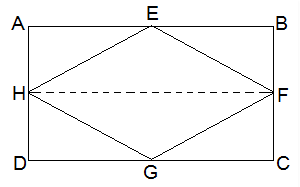# MCQ Questions for Class 9 Maths Chapter 9 Areas of Parallelogram and Triangles with Answers

## MCQ Questions for Class 9 Maths Chapter 9 Areas of Parallelogram and Triangles with Answers

MCQs from Class 9 Maths Chapter 9 – Areas of Parallelogram and Triangles are provided here to help students prepare for their upcoming Maths exam.

MCQs from CBSE Class 9 Maths Chapter 9: Areas of Parallelogram and Triangles

1) If ABCD is a parallelogram, AE ⊥ DC and CF ⊥ AD. If AB = 10 cm, AE = 6 cm and CF = 5 cm, then AD is equal to:
a. 10cm
B.6cm
c. 12cm
d. 15cm

Explanation: Given,

AB = CD = 10 cm (Opposite sides of a parallelogram)

CF = 5 cm and AE = 6 cm

Now,

Area of parallelogram = Base × Altitude

CD × AE = AD × CF

10 × 6 = AD × 5

2) If E, F, G and H are the mid-points of the sides of a parallelogram ABCD, respectively, then ar (EFGH) is equal to:
a. 1/2 ar(ABCD)
b. ¼ ar(ABCD)
c. 2 ar(ABCD)
d. ar(ABCD)

Explanation: Join H and F as shown in the below figure:AH || BF and and DH || CF

AH = BF and DH = CF (H and F are midpoints)

∴, ABFH and HFCD are parallelograms.

ΔEFH and llgmABFH, both lie on a common base, FH.

∴ area of EFH = ½ area of ABFH — 1

area of GHF = 1/2area of HFCD — 2

Adding eq. 1 and 2 we get;

area of ΔEFH + area of ΔGHF = ½ (area of ABFH + area of HFCD)

ar (EFGH) = ½ ar(ABCD)

3) If P and Q are any two points lying on the sides DC and AD respectively of a parallelogram ABCD, then:
a. ar(APB) > ar(BQC)
b. ar(APB) < ar(BQC)
c. ar(APB) = ar(BQC)
d. None of the above

Explanation: ΔAPB and parallelogram ABCD lie on the same base AB and in-between same parallel AB and DC.

ar(ΔAPB) = ½ ar(parallelogram ABCD) — 1

ar(ΔBQC) = ½ ar(parallelogram ABCD) — 2

From eq. 1 and 2:

ar(ΔAPB) = ar(ΔBQC)

4) If ABCD and EFGH are two parallelograms between same parallel lines and on the same base, then:
a. ar (ABCD) > ar (EFGH)
b. ar (ABCD) < ar (EFGH)
c. ar (ABCD) = ar (EFGH)
d. None of the above

5) A median of a triangle divides it into two
a. Congruent triangles
b. Isosceles triangles
c. Right triangles
d. Equal area triangles

Explanation: Suppose, ABC is a triangle and AD is the median.

∴ It will divide ΔABC into two triangles of equal area.

∴ ar(ABD) = ar(ACD) — (i)

also,

ED is the median of ΔABC.

∴ ar(EBD) = ar(ECD) — (ii)

Subtracting (ii) from (i),

ar(ABD) – ar(EBD) = ar(ACD) – ar(ECD)

⇒ ar(ABE) = ar(ACE)

6) In a triangle ABC, E is the mid-point of median AD. Then:
a. ar(BED) = 1/4 ar(ABC)
b. ar(BED) = ar(ABC)
c. ar(BED) = 1/2 ar(ABC)
d. ar(BED) = 2 ar(ABC)

Explanation: See the figure below:ar(BED) = ½ BD.DE

AE = DE (E is the midpoint)

BD = DC (AD is the median on side BC)

DE = ½ AD —- 1

BD = ½ BC —- 2

From eq. 1 and 2, we get;

ar(BED) = (½ ) x (½) BC x (½) AD

ar(BED) = (½) x (½) ar(ABC)

ar(BED) = ¼ ar (ABC)

7) If D and E are points on sides AB and AC respectively of ΔABC such that ar(DBC) = ar(EBC). Then:
a. DE is equal to BC
b. DE is parallel to BC
c. DE is not equal to BC
d. DE is perpendicular to BC

Explanation: ΔDBC and ΔEBC are on the same base BC and also having equal areas.

∴ they will lie between the same parallel lines.

∴ DE || BC.

8) If Diagonals AC and BD of a trapezium ABCD with AB || DC intersect each other at O. Then,
a. ar (AOD) = ar (BOC)
b. ar (AOD) > ar (BOC)
c. ar (AOD) < ar (BOC)
d. None of the above

Explanation: △DAC and △DBC lie on the same base DC and between the same parallels AB and CD.

ar(△DAC) = ar(△DBC)

⇒ ar(△DAC) − ar(△DOC) = ar(△DBC) − ar(△DOC)

⇒ ar(△AOD) = ar(△BOC)

9) If Diagonals AC and BD of a quadrilateral ABCD intersect at O in such a way that ar(△AOD) = ar(△BOC). Then ABCD is a:
a. Parallelogram
b. Rectangle
c. Square
d. Trapezium

Explanation: ar(△AOD) = ar(△BOC)

ar(△AOD) = ar(△BOC)

⇒ ar(△AOD) + ar(△AOB) = ar(△BOC) + ar(△AOB)

Areas of △ADB and △ACB are equal.

Therefore, they must lie between the same parallel lines.

Therefore, AB ∥ CD

Hence, ABCD is a trapezium.

10) If a triangle and a parallelogram are on the same base and between same parallels, then the ratio of the area of the triangle to the area of parallelogram will be:
a. 1:2
b. 3:2
c. 1:4
d. 1:3